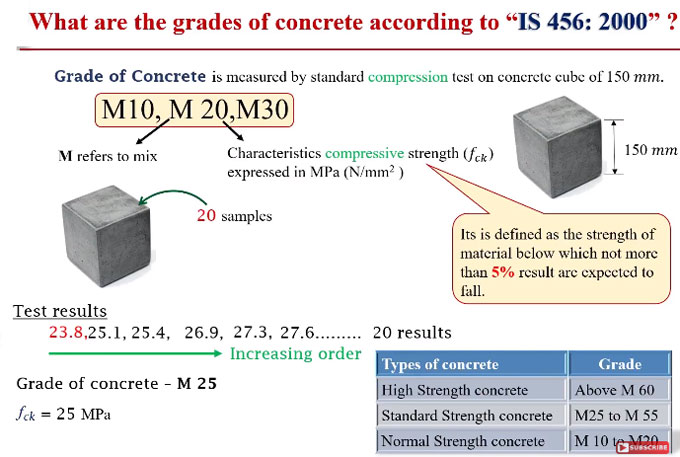# How to determine grades of concrete following IS 456:2000 standardThis construction video tutorial explains the grades of concrete with adherence to IS 456:2000 as well as process for detecting the characteristics strength of concrete.

IS 456-2000 refers to an Indian standard code of practice for general structural use of plain and reinforced concrete.

Grade of concrete determines the compressive strength of concrete. To calculate grade of concrete, standard compression test is carried out on concrete cube of 150 mm. Grade of concrete is demonstrated with M10, M20, M30 etc. where M stands for Mix i.e. mix of constituents used for concrete. Numeric figure in M stands for characteristics compressive strength (fck) that is stated MPa (N/mm2).

Characteristics compressive strength means the strength of material under which not over 5% result is presumed to fall.

As for example if 20 samples of concrete cubes are casted and carried out compression tests for them in the laboratory to determine their compressive strength. Results for test are 27.3, 25.4, 26.9, 25.1, 23.8, 27.6……20 results. If these are organized in increasing order, here 23.8 is less than 25 and remaining are greater than 25 in MPa. So, it signifies that one result falls under 25 MPa and it is detected that only 5% of results fall under 25. Therefore, grade of concrete remains M25.

As per IS code, there are three comprehensive categories for concrete as follow :-

 Type of concrete Grade High Strength Concrete Over M 60 Standard Strength Concrete M25 to M55 Normal Strength Concrete M10 to M20

For online demonstration, watch the following video.